Intro to Programming: Functions and Methods

In this article in my Intro to Programming series, we're going to build some intuition around functions and methods. Specifically, we'll look at what they are, how they work and why we need them.
By Ciprian Stratulat • Jan 11, 2022

Hello, and welcome back to a new article in my Intro to Programming series. Today, we’re going to take a look at functions and methods.

Functions & Methods

In this article, we're going to build some intuition around functions and methods. Specifically, we'll look at what they are, how they work and why we need them.We've already seen some functions. The print function is one example, and it's used for outputting strings to your screen.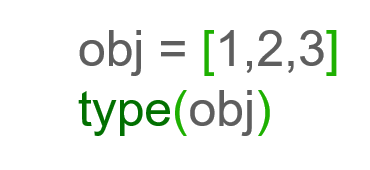Type is another function, and this one takes an object, for example a list, and tells us what its data type is.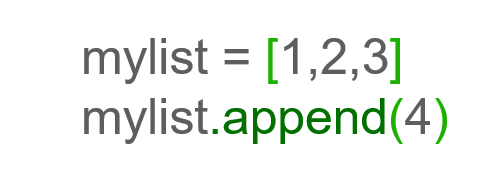We also saw a few examples of methods. Methods are also functions, except you can think of them as being attached to an object. So for example, all list objects have an append method attached to them and we can use that method to add another item to the list.

Now, these two names, functions and methods, may be a bit intimidating, particularly if you associate them with math and you have bad memories of math. The idea behind them, however, is much simpler than the concepts we encountered in math.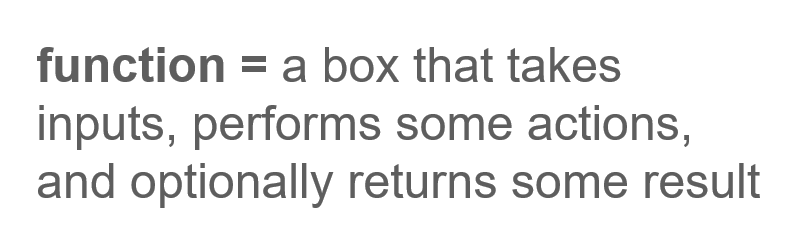You can think of a function as a box that takes some inputs - these can be strings, integer numbers, whatever you want - then performs some actions, typically using those inputs that you provided, and in the end, optionally, returns some result.
The reason I say it optionally returns some results is because a function doesn't necessarily have to give us anything back.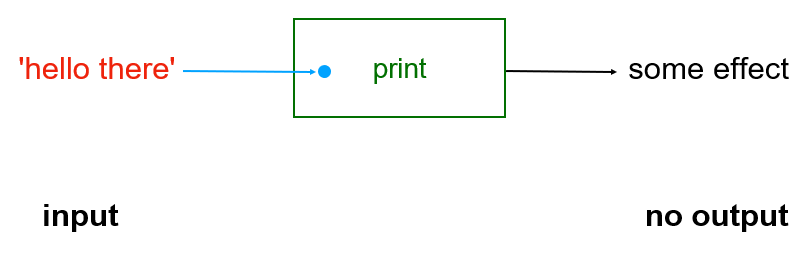For example, let's take the print function. You can picture it as this green box right here. It has a name, print, and it accepts one input, which I drew as this blue dot. You can picture this input as perhaps a hole in the box. Through this hole, we can insert something in the box. Let's say into this blue input hole we insert a piece of paper that has the string hello there on it. This particular box doesn't have an output hole. Inside it, there's a mechanism that performs some actions and then in the end we only observe the effects of that mechanism, which is that the string hello there magically appears on our computer screens.

I think this is a good analogy for functions. But some of these boxes, or let's call them functions from now on, do have an output hole as well.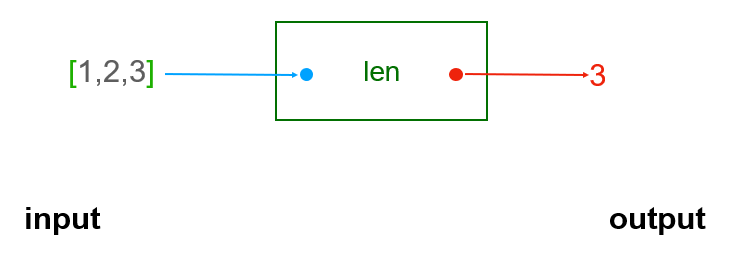Take len, for example. As we saw earlier, it's a built-in function that takes as an input some object and gives us a measure of the length of that object. So if the object is a string, it will give us the number of characters in the string. If the object is a list, it will give us the number of items in the list. The analogy is the same. If we insert a piece of paper into the blue input hole with our list on it, some magic happens inside that box, and out of the red output hole we see a piece of paper with the number 3 on it.

Now, perhaps you're wondering, why do we have functions? They're useful because we can use them to either achieve some effects (such as printing something on the screen), or get the answer to some questions (such as how long is the string hello). But more importantly, functions act a bit like shortcuts.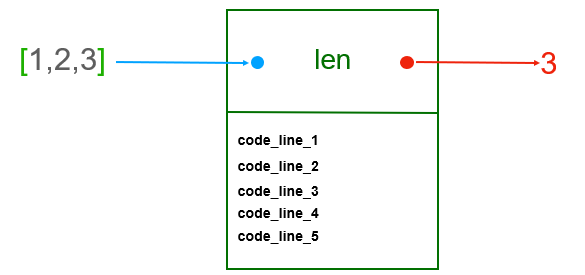If we take the len function I mentioned earlier, you can imagine that, inside that box, there are actually many lines of code that work hard to count whatever we may drop down the input hole. You can see in this diagram, code line 1, code line 2, etc. It doesn't matter right now what each line does, what matters is that there are a bunch of them. Maybe there are only 5 lines, maybe there are 50.

If we didn't have this box right here, this len function, whenever we wanted to count stuff, we would basically have to write out all those lines of code that actually achieve the counting. That's a problem for two reasons. First, we'd have to type a lot more - and by now you know, more code means more typos, and so possibly more problems, so we want to keep our code as short as possible. Secondly, without the concept of a function, we'd have to do a whole lot more work if we have to change something.

Think about it this way. What if we write a program where we need to count 10 different things at various points in the program? We'd have to repeat each one of those lines 10 times. And what if, once we're done, we realize we forgot something? Now we have a lot of places where we need to make changes.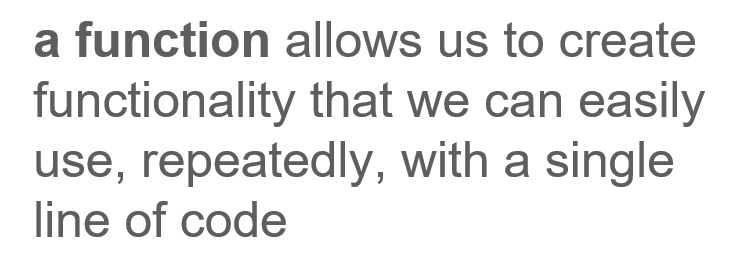So, functions allow us to create functionality that we can then easily use many times, with a single line of code. This is key. A very efficient programmer will look at their code and, whenever they see identical lines of code being repeated throughout the program, they see opportunities to create functions and simplify their code. Once that function is created, it can be used as many times as needed, so in a sense, the more higher-level functions you write, the easier and faster it becomes to do things with code.

Anatomy of a Function

We built some intuition around the concept of a function. We compared it to a box, with separate holes for inputs and outputs. Now, we'll talk about how to build our own boxes. So far, we've only used built-in functions, so we're going to learn how to build our own custom functions.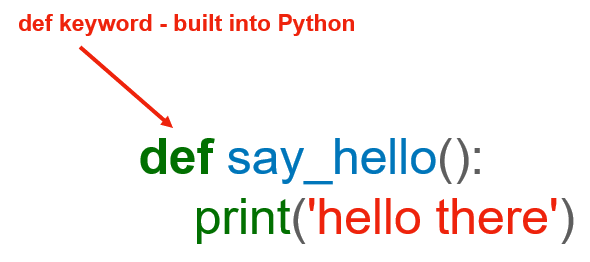We're first going to talk about the syntax for defining a function.

Here we have a very simple function called say_hello. We define functions using the def keyword, which, of course, is short for define,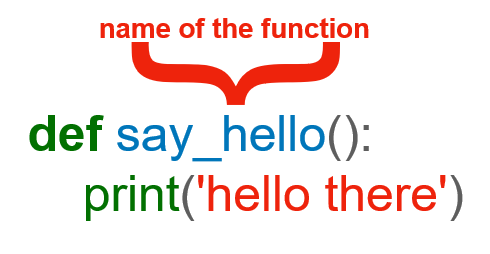and the def keyword is followed by the name of the function. The same restrictions that apply to variable names also apply to function names. My advice to you is to get in the habit of giving your functions names that make sense. That is, names that kinda suggest what the function does. For example, it would be terrible to name this function "function_one" or "penguin". Neither of those names tells me anything about what the function does, and reading code that has penguins in it makes no sense.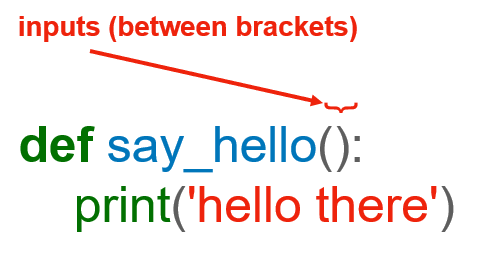After the name of the function, we have these two brackets. Inside them, we'll list any inputs that the function takes. In this case, the say_hello function has nothing inside the brackets, so it takes no inputs. The brackets are still required even if you have no inputs, so it's very important to put them there. We'll go over an example of a function that does take inputs in just a moment.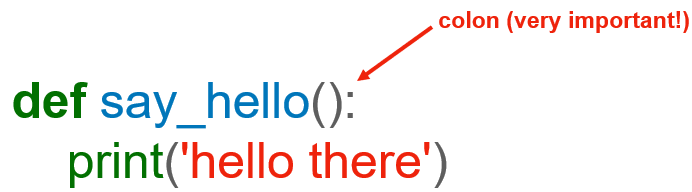Finally, on this line, just like we saw with if-statements and for loops, we have a colon. It serves a similar role: it tells the Python interpreter that the body of the function is following next.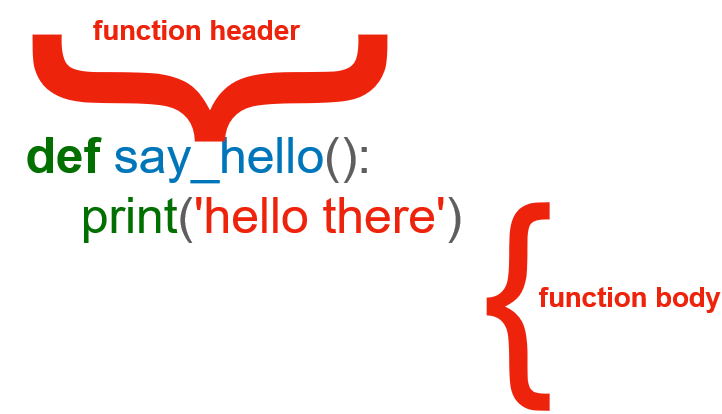This whole first line is the function header. This is where we specify the name of the function and the inputs it takes. What follows below are the internals of the function, or the function body. This is where we write the code that the function will execute when it runs.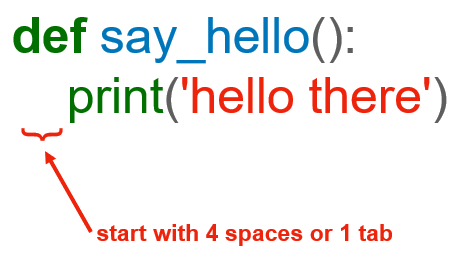The important thing to remember about the function body is that it starts with the same 4 spaces indentation (or 1 tab, if you prefer) that we saw with if-statements and for loops. The indentation is required by Python. Otherwise, it would have no way of telling which lines of code are inside the function and are, therefore, part of it, and which are outside of it.

Functions With Input

Now let's go over another example. This say_hello function is pretty good, but it doesn't do much. What if we wanted to have a custom greeting, depending on a customer's first name?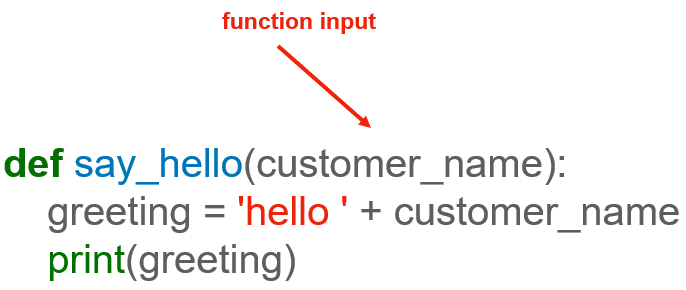We can do this by updating our function, like this.

So not much has changed here. The only thing is that now, between the brackets in the function header, we have this customer_name. This is the input name. The say_hello function now accepts an input and the name of that input is customer_name. You can name your inputs whatever you want - they are there just as placeholders, to represent the values that will get passed to the function. However, as with variables, please choose names that make sense, and that inform the reader of your code what the inputs represent.

The body of the function is also pretty simple. We define a variable called greeting and we set it to the string that consists of the word hello with the customer_name appended at the end, and then we print the greeting.

Functions that Return a Value

So far we have explored only functions that have some effect on the world, but don't actually return a value to us. We mentioned earlier that an example of a function that takes some input and returns an output is len. Now, let's build our own function that takes some input and returns an output.

What might be an example of such a function?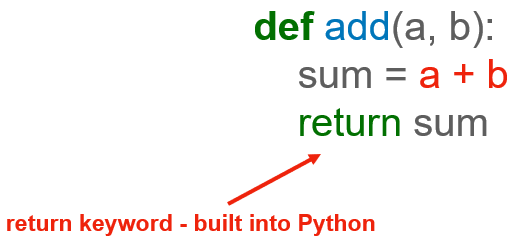A simple one would be a function that takes two numbers, let's say a and b, and returns their sum. Here's the code for it. It looks pretty much like the other functions. It starts with the def keyword, followed by the name of function, which is add. You will often find functions named as verbs or at least containing verbs. That's because we want to indicate in the function name what the function actually does.

So this function named add has two inputs represented by a and b here. You could also call them first_number and second_number, it's up to you. And that's pretty much it for the function header - except, of course, don't forget about the colon at the end. As for the body, most of it should also hopefully be fairly straightforward. We define a variable called sum and we set it to be a plus b.

The last line is where the magic happens. Instead of printing, we write return sum. That return is a keyword and it tells Python that what this function should do is expose the value of the variable sum as an output value.

How a Function Ends

A very important thing to remember about variables defined inside functions is that they are NOT available outside the functions where they are defined. A function is a bit of a black box - the outside world can only see what the function decides to expose via outputs, nothing more. So, for example, code running outside of this function would not be able to inquire about the value of the variable sum directly, unless we use the keyword return to expose that value.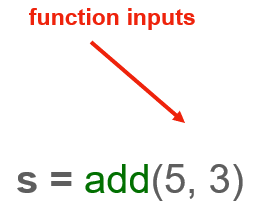To understand how that happens, consider this line of code. Here, we call (or run, or execute, whichever verb you prefer) the function add and we pass it two inputs: 5 and 3. Function inputs are called parameters or arguments. There's a distinction between when you use the word parameters vs the word arguments, but not everyone always makes it. We'll talk more about that in a second. Now, back to our add function, we call the add function and we pass it the integer numbers 5 and 3 as inputs.

As you can see here, in order to capture the result, that is to capture what this function returns, we simply assign the result of the function call to a variable. In this case, we store the result of running the add function in the variable named s.

I want to bring your attention to a few things here. First, when you run a function, you don't need the colon sign at the end because there's no function body following. Also, obviously, there's no def keyword involved either. That's only used for defining a function.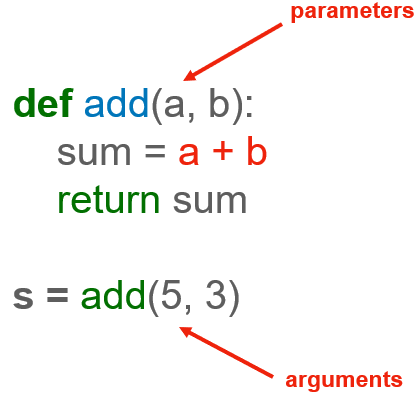Before we start writing some code, let's quickly clarify the difference between parameters and arguments. Parameters are the variables used in the function definition. Arguments are the actual data that you pass to the function when you execute it. As I mentioned, some people will use the two words interchangeably, even though technically that's not correct. It's not entirely crucial right now that you understand and memorize the distinction, but I just wanted you to be aware of it because you'll probably come across it. So, when someone says parameters, they're referring to variables inside a function definition. When they talk about arguments, they talk about the actual numbers, strings, lists etc. that are passed to the function when they execute it.

Now we're going to get hands-on and start writing some code to explore functions in greater detail.

Defining a Function

Let's start by writing our initial say_hello function: def say_hello(): print('hello there'). Now, we can run this function by typing say_hello(). Again, notice that even if we don't have any inputs, we still have to add the parentheses. What happens if we leave them out? Well, Python evaluates the name say_hello and concludes that it's a function with the name say_hello. But it does not actually run it. To run a function, you need the parentheses.

# Let's define our function
def say_hello():
print('hello there')

# and now we'll run it
say_hello()
# Our output will be hello there

# If we forget to add our parentheses
say_hello
# we get the output: <function __main__.say_hello>
# Don't forget the parentheses!

Let's also write our more advanced example, where we print a custom message: def say_hello(customer_name): greeting = 'hello ' + customer_name print(greeting). Remember, when we merge strings using the plus operator, you have to add a space before the quotation mark if you want a space between the strings. Notice that this function has the same name as the one above, so it basically overwrites it. If we just run say_hello() like before, it will tell us we're missing a value for customer_name. That makes sense, since we updated the definition for say_hello to require the customer name as an input.

# Let's update our function
def say_hello(customer_name):
greeting = 'hello ' + customer_name
print(greeting)
# Notice the space after hello and before the '

# If we try to run it
say_hello()
# We get an error code:
# ---------------------------------------------------------------------------
#TypeError                                 Traceback (most recent call last)
#~\AppData\Local\Temp/ipykernel_27592/2814888808.py in <module>
#----> 1 say_hello()
#
#TypeError: say_hello() missing 1 required positional argument: 'customer_name'

This error message might be a bit cryptic at first, but the gist of it is that we're trying to run the say_hello function, but it requires an input - some value to be assigned to the customer_name variable. So let's actually call it with an input. say_hello('Sam'), for example will return hello Sam. say_hello('Lucy') will print hello Lucy and so on.

say_hello('Sam')
# Our output will be hello Sam

say_hello('Lucy')
# Our output will be hello Lucy

You can also, of course, call this function in a loop. For example, if we run for name in ['Sam', 'Lucy', 'Computer']: say_hello(name), we'll get a greeting for each name in our list, so hello Sam, hello Lucy, hello Computer.

# Let's create a loop by inputting a list
for name in ['Sam', 'Lucy', 'Computer]:
say_hello(name)
# Our output will be hello Sam, hello Lucy, hello Computer

If you are following along in a Jupyter notebook, you can easily rerun a cell using shift+enter, so let's use that to update that greeting to include proper capitalizations. Let’s go into the cell where we defined our say_hello() function, change the greeting, and then re-run the code in the cell by pressing shift+enter. Then, when we come back to the cell that contains the for loop, we can click inside it, press shift and enter at the same time, and we'll see the updated output.

# Let's fix our function to use proper capitalization
# Change the cell to say
greeting = 'Hello ' + customer_name
# Then press shift and enter to rerun the code
# When we rerun our list, we will get
# Hello Sam, Hello Lucy, Hello Computer

Functions With Default Values

I want to show you one more thing about function parameters. They can actually have a default value. So far, we've only seen instances where the parameter was required. The say_hello function requires that we pass an argument for the customer_name when we want to run the function. If the argument isn't passed, we'll get an error.

But there are situations where you want to write functions that have parameters with default values. Let me show you an example. Let's generalize this say_hello function. Let's say that we are writing an automatic marketing platform, and we need a way to greet our customers, who are in different parts of the world. We can't just use the word Hello - that will only work with our customers who speak English. But let's also say that most, though not all, speak English. In that case, it would make sense to set hello to be the default greeting, but we would also want to have a way to specify a different greeting in some cases.

We can achieve that using a default parameter. Let me show you. Let's rewrite the say_hello function to be def say_hello(customer_name, greeting_word='Hello '): greeting = greeting_word + customer_name print(greeting). What did we do here? Well, the function looks pretty similar to what we had before, except we now have a second parameter, named greeting_word, and we assign to that parameter the default value Hello. This is our default parameter. So now, our greeting is made up of the greeting_word and the customer_name.

# Let's rewrite our function with an added parameter
def say_hello(customer_name, greeting_word='Hello '):
greeting = greeting_word + customer_name
print(greeting)

Let's call this function. If we call it like before, say_hello('Sam'), the output is hello Sam. Notice that we didn't specify a greeting_word, only a customer_name, so our greeting word was set to the default Hello. What if Sam is French? We can then instead execute say_hello('Sam', 'Bonjour ') and we see that the greeting is now bonjour Sam. I added an extra space after bonjour so that there would be a space between bonjour and Sam. Remember that when we merge strings using the plus operator, a space is not added by default, so you have to add one yourself if you want one.

# Let's call our function
say_hello('Sam')
# Our output will be Hello Sam

# Let's adjust our greeting word and run it again
say_hello('Sam', 'Bonjour ')
# Our output is now Bonjour Sam

Default parameters are good to know and use. Lots of built-in Python functions have default parameters, and you can discover them using the Python documentation.

Next, let's go over a few examples of functions that return results. A very basic one that we saw earlier is a function that adds two numbers. Let's write that: def add(a, b): sum = a + b return sum. Now, we can call that function, let's say result = add(5,3). Notice that nothing got printed on the screen this time, but, if we print(result), we get 8. What happened here is that we executed the add function with two arguments, the integer 5 and the integer 3, and the result of that execution got stored in the variable named result. We then printed the value stored in result, and we got 8. Hopefully that makes sense.

# Let's define our function
sum = a + b
return sum

# If we call our function
# nothing is printed in the console

# Let's try the print function
print(result)
# Our output is now 8

What happens if I accidentally run result = add(0)? Well, we get an error - and we've seen this error before. Basically, Python saw the integer number 0 and assigned that in the place of a, but saw nothing for b because we didn't pass a second argument. Try calling the add function again. Let's say res = add(0, 5). Now, when we print(res), we get 5.

# Let's see what happens when we run
# We get the following error:
#---------------------------------------------------------------------------
#TypeError                                 Traceback (most recent call last)
#~\AppData\Local\Temp/ipykernel_27592/2149202480.py in <module>
#
#TypeError: add() missing 1 required positional argument: 'b'

# Let's fix our code by adding a second integer
print(res)
# Our output is now 5

Perhaps you're wondering - why not just print the result directly instead of assigning it to this variable? Well, we could do that, of course, and in this case it would work the same. However, oftentimes we define functions that compute some sort of intermediary value that we need to reuse throughout our program. We need a way to store that value so we can reuse it later. In that case, printing won't help you. Printing a value does not store it in memory anywhere - it's printed, and then it's gone. If you want to store the result of some function execution, you need to define the function in such a way that it returns a result using the keyword return and, when you execute that function, you need to assign the result to some variable.

Using Return in a Function

One more thing: you can only use return once inside a function, and only as the last line of the function's code. Once the keyword return is reached, the function terminates and the result is returned. Let me show you. Let's modify our add function, and add a print function after the return. So now we have def add(a, b): sum = a + b return sum print('hey'). And now let's write res = add(2,2). Notice something? The word hey was not printed. We know that the function executed successfully, because if we print(res) now, we get 4, which is indeed the result of 2 + 2. But the word hey was not printed. Why is that? It's because, once the code execution reaches the keyword return, the function terminates.

# Let's update our add function
sum = a + b
return sum
print('hey')
# and run it
# Nothing is printed in the console

# Let's check our result
print(res)
# Our output is now 4

Compare that with this: def add(a, b): sum = a + b print('hey') return sum. Here, we're printing hey before we return the result. So now let's write res = add(2,2) and if we run this, we see that the word hey was printed. Not only that, but, of course, if we print(res) now, we see that res was also updated with the correct result value. So, to sum up, once the execution of a function reaches the return keyword, the function terminates.

# Let's update our code again
sum = a + b
print('hey')
return sum
# Now when we run the code
# Our output is 'hey'

print(res)
# Our output is 4

Functions With Multiple Exit Points

So far, we've only built functions with a single exit point. Some code gets executed, and a single possible output gets returned or printed at the end. Next up, we'll look at functions that have more than one exit point.

There's one more thing you need to be aware of when it comes to using the return keyword in Python. While you can only execute a single return statement in a function, you can still have multiple exit paths possible inside that function. Let me explain.

For example, let's consider two integers representing the dollar amount of two recent purchases that a customer made. We want to write a function that takes the two numbers as inputs and checks whether the customer has a high balance. We can define a high balance as amounts greater than 1000 dollars. We can write the following solution. First we define the function, let's call it has_high_balance, so: def has_high_balance(a, b):. a and b here represent the dollar amounts of the two purchases that the customer made most recently. Next, we calculate their sum, so sum = a + b. Now, we need to check if that sum is greater than 1000, so if sum > 1000: return True. What this means is that our function will return the boolean value True if the sum of the two purchases is higher than 1000 dollars. In other words, our function will return True if the customer has a high balance. Next, we write the else branch, so else: return False. So now, if the sum is not greater than 1000, we return False.

# Let's define our function
def has_high_balance(a, b):
sum = a + b
if sum > 1000:
return True
else:
return False

Let's go ahead and run this and check that it works. We can define a variable named is_high_balance and we'll first set it to the result of calling the has_high_balance function with 400 and 500 as inputs. So is_high_balance = has_high_balance(400, 500). If we now print(is_high_balance), we get False and that makes sense because 400 + 500 is 900, which is less than 1000. Let's do this again, this time is_high_balance = has_high_balance(1000, 200). If we print(is_high_balance) now, we get True, because 1000 + 200 is 1200, which is greater than 1000, so the customer is running a high balance.

# Let's run our function with 400 and 500
is_high_balance = has_high_balance(400, 500)
# and print it
print(is_high_balance)
# Our output is False

# Let's try 1000 and 200
is_high_balance = has_high_balance(1000, 200)
# and print
print(is_high_balance)
# Our output is True

This is not the shortest or the prettiest implementation of our function, but I wrote the solution this way to show you that you can have multiple return keywords in a function if they correspond to different exit points out of the function. In this case, if the sum is greater than 1000, we return some value, in this case the boolean value True, and if the sum is not greater than 1000, we return a different value, in this case the boolean value False. If a function has an if-statement inside it, it is pretty common to have multiple return statements, one per branch typically.

Let’s wrap up our exploration of functions by clarifying a couple of important points.

Nested Functions

The first point is that a function can actually call other functions inside it. There's nothing preventing us from doing that. In fact, it's very common. The second one has to do with how function execution affects the order in which particular lines of code are executed.

Let's revisit our high_balance function. See the line where we calculate the sum? We could replace that with a call to our add function that we wrote above. So the code now becomes: def has_high_balance(a, b): sum = add(a,b) if sum > 1000: return True else: return False. It looks very similar to what we had earlier, except instead of using the plus operator to add the numbers a and b, we call the add function that we defined previously. This is totally valid code.

# Let's update our function
def has_high_balance(a, b):
if sum > 1000:
return True
else:
return False

Let's run it again to check it. So again, we run is_high_balance = high_balance(1000, 200). And we see that the word hey got printed. If you look above at our last definition for the add function, you can see that we are printing the word hey before we return the value. We probably don't need to do that, but that's what we had our add function do, so that's ok. Now, if we print(is_high_balance) we again get True, which makes sense because 1000 + 200 is 1200 which is greater than 1000. So functions can actually call other functions inside their definition, and this is very powerful because it allows for code reuse.

# Let's run our function with 1000 and 200 again
is_high_balance = has_high_balance(1000, 200)
# Our output is hey because of our add function

# Let's print the result
print(is_high_balance)
# Our output is True

Functions and Execution Order

Finally, there's one last point that I want to insist on. That is that the function execution affects the order in which particular lines of code are executed. Let me show you what I mean by that. I'm going to write a slightly longer piece of code, and we'll go over it in just a second. See if you can figure out what this program does before we go over it.

Ok, so before we go over this little program, let’s toggle the line numbers for this cell. We can easily do this by clicking the keyboard icon in the menu bar of our Jupyter notebook, searching for the word 'line', and clicking on 'toggle line numbers'. The keyboard icon shows us all the commands that we can run in our Jupyter notebook and their corresponding keyboard shortcuts. If you use Jupyter notebook a lot, I encourage you to memorize some of the shortcuts that you use all the time because it's going to make you a faster programmer.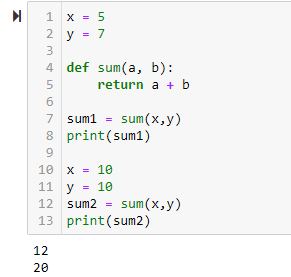We can see some nice line numbers on the side here. So, what does this program do? Let's go line by line. On line 1, we define a variable x, and we assign it the integer value 5. On line 2, we define a variable y, and we assign it the integer value 7. On lines 4 and 5, we define a function called sum that takes two integer numbers and returns their sum. On line 7, we define a variable called sum1, and we assign it the result of executing the sum function with the input values stored in variables x and y.

Let's focus a bit on this line 7. When our program execution reaches this line, what happens next is that the Python interpreter figures out that the sum function is defined on line 4 above, so it jumps to that line, sets a to be whatever is stored in our variable x, which is 5, and then sets b to whatever is stored in our y variable, which is 7, then goes to line 5, calculates a + b, so 5 + 7, which is 12, and returns 12. Then, it jumps back to line 7 and assigns 12 to the variable named sum1. Following that, normal execution resumes, so the next code line that it runs is line 8, which prints sum1, so it prints 12.

Next, it executes line 10, where it updates x and sets it to the integer 10. Next, the program execution runs line 11, where it updates y and sets it to the integer 10. Next, on line 12, it sees that you are again running the sum function. It again figures out that the function is defined on line 4 above, so it jumps to that line, sets a to be whatever value we have in x, which now is 10, and then sets b to whatever value we have in y, which is also 10, then goes to line 5, calculates a + b, so 10 + 10, which is 20, and then returns 20. Then, it jumps back to where it was before, to line 12, and assigns the value 20 to the variable named sum2. Following that, once again, normal execution resumes, so the next code line that runs is line 13, where the program prints the value stored in sum2, which is 20.

If you run this code, you'll see that the outputs are indeed 12 and 20. So, when you define a function, the function is not actually run. A function definition is the act of creating the function, not of using that function. A function definition is just a way to tell the Python interpreter “hey, I made this box that takes these inputs, does this magic thing and returns this result, and I want you to be aware that this box exists because I'm going to use it at some point in the future”. So on line 4 and 5, you just define your function and you tell the Python interpreter about it.

Following that, code lines are executed in normal order, top to bottom, one after the other, until we hit a function execution. On line 7, the Python interpreter sees that you are trying to run the function that you created earlier, so it basically jumps back to the function body. Then, after it assigns to each parameter the values that you passed to the function, it runs the code lines inside the function body one by one, in order. Once the function finishes, it returns to where it left off, which, in this case, is line 7, and then again continues line by line. So the important thing to remember here is that after a function is run, the program execution returns to the specific line that called the function.

That's all for now as far as functions are concerned. Functions are incredibly powerful building blocks, and you'll use them heavily, so spend some time practicing writing them and using them. In addition to the practice exercises we provide, you can also design your own by challenging yourself to write your own functions and then running them with different parameters.Ciprian Stratulat

Ciprian is a software engineer and the CTO of Edlitera. As an instructor, Ciprian is a big believer in first building an intuition about a new topic, and then mastering it through guided deliberate practice.

Before Edlitera, Ciprian worked as a Software Engineer in finance, biotech, genomics and e-book publishing. Ciprian holds a degree in Computer Science from Harvard University.